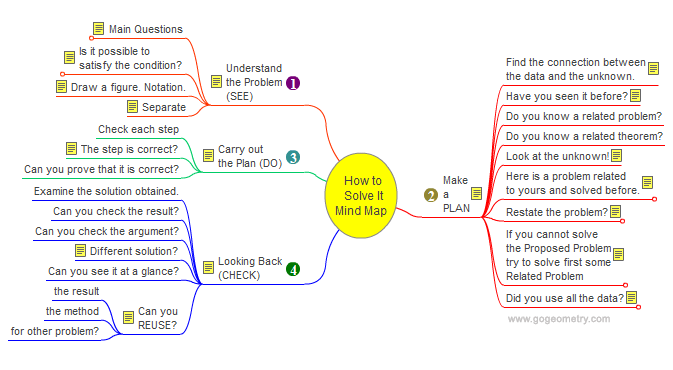Problem 689: Triangle, Three Excircles, Tangency points, Tangent lines, Concurrent Lines, Mind Map, Polya. Level: High School, College, SAT Prep. The figure shows a triangle ABC with the excircles EA, EB, and EC tangent to the extension of sides at the points A1, A2, B1, B2, C1, and C2. Lines BC1 and CB2 meet at A3, CA1 and AC2 meet at B3, AB1 and BA2 meet at C3. Prove that lines A3A, B3B, and C3C are concurrent.Geometry problem solving Geometry problem solving is one of the most challenging skills for students to learn. When a problem requires auxiliary construction, the difficulty of the problem increases drastically, perhaps because deciding which construction to make is an ill-structured problem. By “construction,” we mean adding geometric figures (points, lines, planes) to a problem figure that wasn’t mentioned as "given." How to Solve It, Interactive Mind Map George Pólya's 1945 book "How to Solve It, A new aspect of Mathematical Method", is a book describing methods of problem solving. It suggests the following steps when solving a mathematical problem: (1) First, you have to understand the problem. (2) After understanding, then make a plan. (3) Carry out the plan. (4) Look back on your work. How could it be better?    Mind Map Help. To see a note: Hover over a yellow note button. To Fold/Unfold: click a branch. To Pan: click and drag the map canvas.Recent Additions